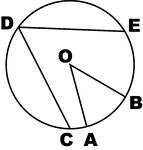### Inscribed and Central Angles

Illustration of circle with inscribed angle and central angle.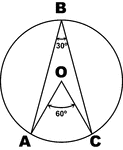### Inscribed and Central Angles 30 60

Illustration of circle with inscribed angle 30 and central angle 60.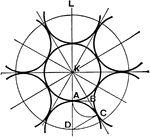### Circle to Circle Tangents

Equal circles inside and tangent to the outside circle, also tangent to each other### Circle Triangle Tangents

Six equal circles tangent to each other and to the sides of the triangle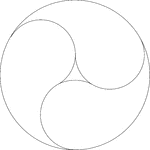### 3 Yin Yang Design Symbols In A Circle

Design made by drawing one large circle and then three circles that are internally tangent to the original…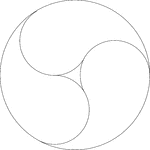### 3 Yin Yang Design Symbols In A Circle

Design made by drawing one large circle and then three circles that are internally tangent to the original…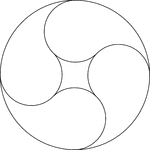### 4 Yin Yang Design Symbols In A Circle

Design made by drawing one large circle and then four circles that are internally tangent to the original…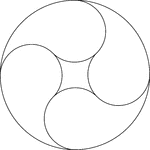### 4 Yin Yang Design Symbols In A Circle

Design made by drawing one large circle and then four circles that are internally tangent to the original…### Circle with Arc, Chord, Inscribed Angle, Circumscribed Polygon

Illustrations of a circle with arc, chord, inscribed angle, and circumscribed about a polygon.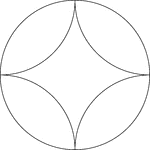### Arcs Inscribed In A Circle

A design created by inscribing 4 congruent tangent arcs in a circle.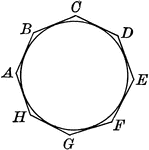### Circle with Circumscribed Polygon

Illustrations of a circle with a circumscribed polygon. Or, a circle inscribed in an octagon.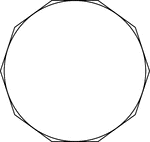### Decagon Circumscribed About A Circle

Illustration of a decagon circumscribed about a circle. This can also be described as a circle inscribed…### Decagon Inscribed In A Circle

Illustration of a decagon inscribed in a circle. This can also be described as a circle circumscribed…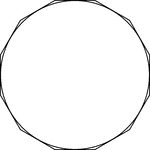### Dodecagon Circumscribed About A Circle

Illustration of a dodecagon circumscribed about a circle. This can also be described as a circle inscribed…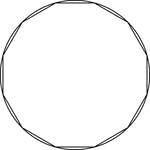### Dodecagon Inscribed In A Circle

Illustration of a dodecagon inscribed in a circle. This can also be described as a circle circumscribed…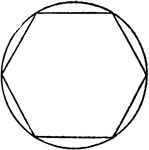### Hexagon Inscribed in Circle

Illustration of hexagon inscribed in circle. Or, circle circumscribed about hexagon.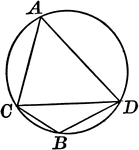### Circle With Inscribed Angle Greater Than Semicircle

Illustration of a circle with an angle inscribed in a segment greater than a semicircle, an acute angle.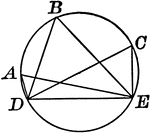### Circle With Inscribed Angle Less Than Semicircle

Illustration of a circle with an angle inscribed in a segment less than a semicircle, an obtuse angle.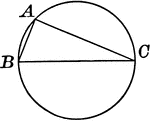### Circle With Inscribed Right Angle in Semicircle

Illustration of a circle with a right angle inscribed in a semicircle.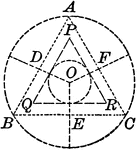### Circle With Triangles and Circle Within

Illustration showing a circle with equilateral triangles and another circle within.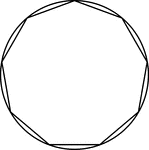### Nonagon Inscribed in a Circle

Illustration of a regular nonagon inscribed in a circle. This could also be described as a circle circumscribed…### Pentagon Inscribed in Circle

Illustration of pentagon inscribed in circle. Or, circle circumscribed about pentagon.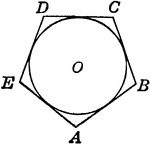Illustration of polygon circumscribed about circle. Or, circle inscribed in the polygon.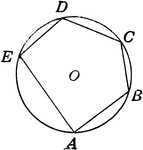### Polygon Inscribed in Circle

Illustration of polygon inscribed in circle. Or, circle circumscribed about the polygon.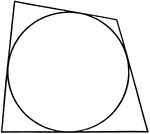Illustration of a quadrilateral circumscribed about a circle. This could also be described as a circle…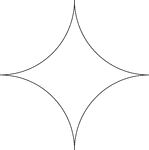### Reflected Arcs Of A Circle

A design created by dividing a circle into 4 equal arcs and reflecting each arc toward the center of…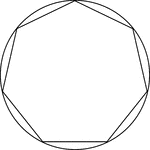### Regular Heptagon/Septagon Inscribed In A Circle

Illustration of a regular heptagon/septagon inscribed in a circle. This can also be described as a circle…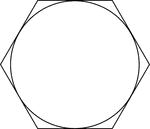### Regular Hexagon Circumscribed About A Circle

Illustration of a regular hexagon circumscribed about a circle. This can also be described as a circle…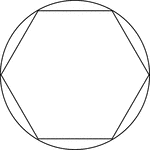### Regular Hexagon Inscribed In A Circle

Illustration of a regular hexagon inscribed in a circle. This can also be described as a circle circumscribed…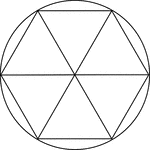### Regular Hexagon Inscribed In A Circle

Illustration of a regular hexagon inscribed in a circle. This can also be described as a circle circumscribed…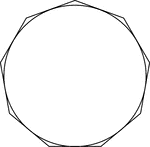### Regular Nonagon Circumscribed About A Circle

Illustration of a regular nonagon circumscribed about a circle. This can also be described as a circle…### Regular Nonagon Inscribed In A Circle

Illustration of a regular nonagon inscribed in a circle. This can also be described as a circle circumscribed…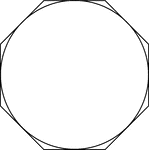### Regular Octagon Circumscribed About A Circle

Illustration of a regular octagon circumscribed about a circle. This can also be described as a circle…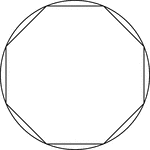### Regular Octagon Inscribed In A Circle

Illustration of a regular octagon inscribed in a circle. This can also be described as a circle circumscribed…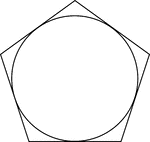### Regular Pentagon Circumscribed About A Circle

Illustration of a regular pentagon circumscribed about a circle. This can also be described as a circle…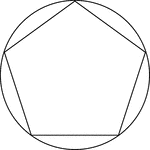### Regular Pentagon Inscribed In A Circle

Illustration of a regular pentagon inscribed in a circle. This can also be described as a circle circumscribed…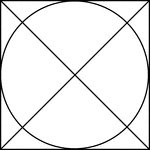### Square Circumscribed About A Circle

Illustration of a square, with diagonals drawn, circumscribed about a circle. This can also be described…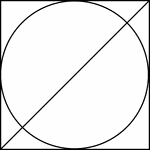### Square Circumscribed About A Circle

Illustration of a square, with 1 diagonals drawn, circumscribed about a circle. This can also be described…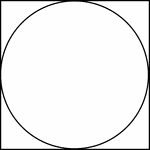### Square Circumscribed About A Circle

Illustration of a square circumscribed about a circle. This can also be described as a circle inscribed…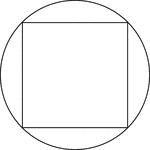### Square Inscribed In A Circle

Illustration of a square inscribed in a circle. This can also be described as a circle circumscribed…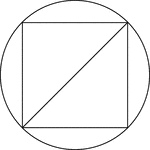### Square Inscribed In A Circle

Illustration of a square inscribed in a circle. This can also be described as a circle circumscribed…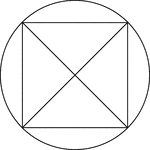### Square Inscribed In A Circle

Illustration of a square, with diagonals drawn, inscribed in a circle. This can also be described as…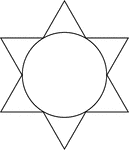### Star Circumscribed About A Circle

Illustration of a 6-point star (convex dodecagon) circumscribed about a circle. This can also be described…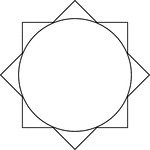### Star Circumscribed About A Circle

Illustration of an 8-point star (convex polygon) circumscribed about a circle. This can also be described…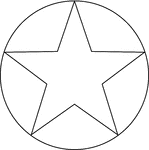### Star Inscribed In A Circle

Illustration of a 5-point star inscribed in a circle. This can also be described as a circle circumscribed…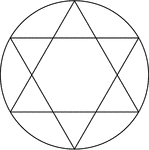### Star Inscribed In A Circle

Illustration of a 6-point star created by two equilateral triangles (often described as the Star of…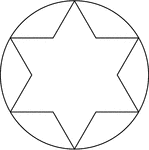### Star Inscribed In A Circle

Illustration of a 6-point star (convex dodecagon) inscribed in a circle. This can also be described…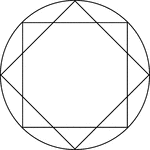### Star Inscribed In A Circle

Illustration of an 8-point star, created by two squares at 45° rotations, inscribed in a circle.…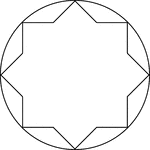### Star Inscribed In A Circle

Illustration of an 8-point star, or convex polygon, inscribed in a circle. This can also be described…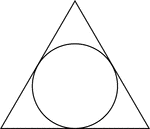### Triangle Circumscribed About A Circle

Illustration of an equilateral triangle circumscribed about a circle. This can also be described as…### Triangle Inscribed in Circle

Illustration of triangle inscribed in circle. Or, circle circumscribed about triangle.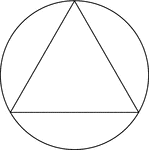### Triangle Inscribed In A Circle

Illustration of an equilateral triangle inscribed in a circle. This can also be described as a circle…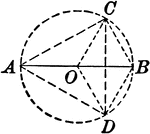### Circle With Inscribed Triangle and Radii

Illustration showing a circle with an inscribed triangle and radii.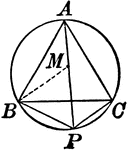### Circle With Inscribed Equilateral Triangle

Illustration showing an equilateral triangle inscribed in a circle.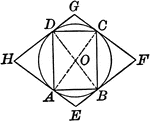### Circle With Inscribed and Circumscribed Quadrilaterals

Illustration showing a circle with an inscribed and circumscribed quadrilateral.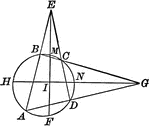### Circle With Inscribed Quadrilateral and Triangles Formed

Illustration showing a circle with an inscribed quadrilateral and triangles formed by extended chords.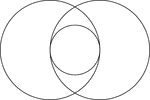### 2 Intersecting Circles

2 congruent circles whose intersection includes a tangent circle with diameter equal to the radii of…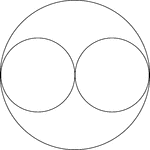### 2 Smaller Horizontally Placed Circles In A Larger Circle

A large circle containing 2 smaller congruent circles. The small circles are externally tangent to each…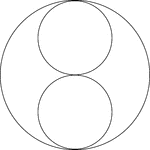### 2 Smaller Vertically Placed Circles In A Larger Circle

A large circle containing 2 smaller congruent circles. The small circles are externally tangent to each…